## Background

Deoxyribonucleic acid (DNA), often called the molecule of life is found in almost all living organisms. According to central dogma of molecular biology, the genetic information contained within DNA in the form of genes is carried by Ribonucleic acid (RNA), which finally gets translated to protein (DNA -> RNA -> Protein).

RNA Sequencing (RNA Seq), a powerful technique which uses high-throughput Next Generation Sequencing (NGS), determines the quantity of RNA present within a biological sample. It further provides information on the expression levels of genes in response to physiological or pathological condition. For example, RNA Seq analysis performed on samples obtained from Covid infected and uninfected individuals sheds information on the human genes which are either up-regulated (higher expression) or down-regulated (lower expression) in response to infection. Identifying these differentially expressed genes (DEG’s) is the key to understand the biological differences between the healthy and diseased state and furthermore provides valuable insights into pathology of the disease. Moreover, the identified DEG’s can serve as candidate biomarkers for diagnostics or as therapeutic targets for treatment.

## Machine learning in RNA-Seq analysis

Differential gene expression analysis yields huge list of genes considered significant in spite of stringent statistical tests and making the search of actual genes a daunting task. There are several challenges associated with this high dimensional data in terms of computational cost and memory. The problem could be overcome in part by utilizing the dimensionality reduction techniques of machine learning algorithms. Dimensionality reduction involves:

1. Feature selection - works by removing the redundant or irrelevant features and selects a subset of existing features without transformation
2. Feature extraction – selects the most relevant features and transforms the features into low dimensional space

Feature selection algorithms are further categorized as:

1. Filter methods – picks features based on correlation to target variable. Independent of any algorithm and generally used as preprocessing step.

Examples: Correlation coefficient, Chi square test, Fisher score

1. Wrapper methods – picks features based on classifier performance. Computationally expensive due to the repeated learning and cross validation.

Examples: Recursive feature elimination (RFE), Boruta, Genetic algorithms

1. Embedded methods - combines the qualities of both filter and wrapper methods.

Examples: Lasso regularization, Decision trees

## Objective

To handle the curse of dimensionality of RNA Seq, two most effective feature selection algorithms - Boruta and RFE have been explored. Both are wrapper algorithms built around random forest classification algorithm. Finally, a random forest model is built with the selected features to eventually aid in the classification of Covid-19. The study can serve two important purposes:

1. DEG’s identified through feature selection can have great implication towards clinical aspects as biomarkers
2. Machine learning models built on selected DEG’s would be much more efficient in classifying Covid-19 than a model built with all the identified DEG’s

## Data Source

Read counts for the study have been obtained from “126 RNA Seq datasets of COVID-19” from Kaggle. The data included counts of 191887 genes for 100 Covid affected and 26 control/healthy samples.

The Original data used in this application is sourced from https://www.ncbi.nlm.nih.gov/geo/query/acc.cgi?acc=GSE157103 by Kaggle and transformed to a usable format. We have kept a zipped file in usable format which has been used in the code below. Kindly download the data from the link by clicking on Covid RNA Sequence Data.

## Workflow

Entire work has been done using “R” environment. Differential expression analysis has been done with Limma Bioconductor package using the Voom method.Figure 1: Workflow for Feature selection of RNA-Seq data for Covid-19 classification

## Installation of dependencies

```        # We have used R version 4.1.0 (2021-05-18) -- "Camp Pontanezen"
# make sure to install Rtools for Building R Packages, not essential but good to have
# Install the Rtools from here https://cran.r-project.org/bin/windows/Rtools/
writeLines('PATH="\${RTOOLS40_HOME}\\\\usr\\\\bin;\${PATH}"', con = "~/.Renviron")
# restart RStudio
# run this command to check
Sys.which("make")
# you should see output like this , "C:\\rtools40\\usr\\bin\\make.exe"
# you can test a installation by running this installation
# install.packages("jsonlite", type = "source") , although not essential
install.packages("jsonlite", type = "source")
install.packages("BiocManager")
BiocManager::install("edgeR")
BiocManager::install("Glimma")
BiocManager::install("biomaRt")
install.packages("gplots")
install.packages("gdata")
install.packages("caret")
install.packages("Boruta")
BiocManager::install("VennDetail")

```

```        library(edgeR)
library(Glimma)
library(limma)
library(biomaRt)
library(ggplot2)
library(RColorBrewer)
library(gplots)
library(gdata)
library(caret)
library(Boruta)
library(dplyr)
library(VennDetail)
library(data.table)
library(DESeq2)

```

## Reading the count data

```    counts <- read.csv("COVID_RNA_Seq.csv")
rownames(counts) <- counts\$X
counts\$X <- NULL
dim(counts)
191887    126

covid001 covid002 covid003 covid004  ...     control023 control024 control025 control026
ENST00000000233  725.157  481.218  722.342 610.7530  ...      3045.0000  1140.6400 2872.11000   1824.930
ENST00000000412    0.000    0.000    0.000   0.0000  ...         0.0000     0.0000    0.00000      0.000
ENST00000000442  164.537  127.731  142.824  76.3582  ...        12.5659    12.4255    3.65413    211.593
ENST00000001008  230.460  135.377  150.590 334.1970  ...       275.6140   429.5400  685.06200    482.911
ENST00000001146    0.000    0.000    0.000   0.0000  ...         0.0000     0.0000    0.00000      0.000
ENST00000002125    0.000    0.000    0.000   0.0000  ...        22.3258     0.0000    0.00000      0.000
```

Table 1: Input data with samples as columns and genes as rows

## Reading experiment summary with sample information

```    info <- read.csv("COVID_RNA_info.csv")
group <- factor(info\$Status)

```

## Data Preprocessing

```    # DGEList object is used by edgeR package to store count data.
counts <- DGEList(counts)

# To check the genes which have zero counts across 126 samples
table(rowSums(counts\$counts==0)==126)

FALSE   TRUE
167220  24667

```

Note that, Around 13% genes have zero counts.

```    # Filtering zero counts and other low expressed genes
keep <- filterByExpr(counts, group=group)
counts <- counts[keep, keep.lib.sizes=FALSE]
dim(counts)
64401   126

```

We note that the counts have reduced from 191887 to 64401.

## Exploratory Data Analysis

### Plotting the library sizes

```    barplot(counts\$samples\$lib.size, names=colnames(counts), las=2, ann=FALSE, cex.names=0.75)
mtext(side = 1, text = "Samples", line = 4)
mtext(side = 2, text = "Library size (millions)", line = 3)
title("Barplot of library sizes")

```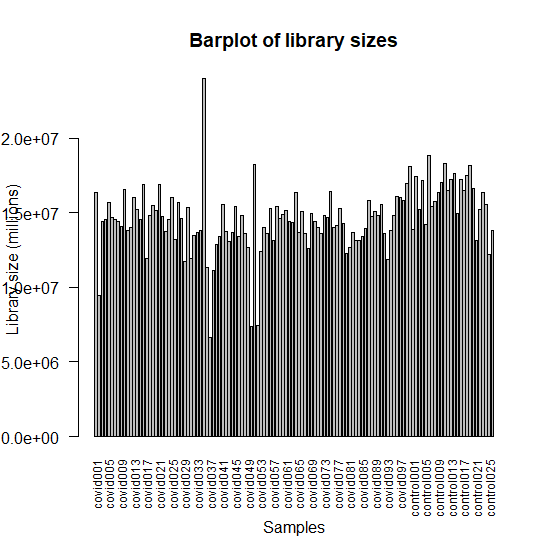Figure 2 : Bar plot of library sizes of samples

### Plotting the log distribution of counts

```    logcounts <- cpm(counts,log = TRUE)
# Log distribution of first twenty samples
boxplot(logcounts[,1:20],xlab="",ylab="Log normalized cpm",las=2)
abline(h=median(logcounts),col='red')
title("Boxplot of Log normalised cpm")

```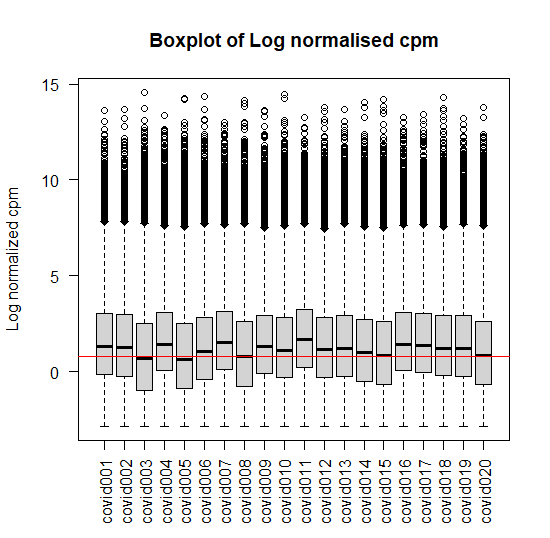Figure 3: Box plot of Log normalised cpm of first twenty samples

### Multidimensional scale plotting

```    # PCA analysis to determine the source of variation in data
labels <- paste(info\$Sample.name, info\$Status)
glMDSPlot(counts, labels=labels, groups=group, folder="mds")

```

Figure 4: MDS plot of dimensions 1 & 2 and Variance of different dimensions

## Normalization

```    # Generate normalisation factors to be used downstream
countdata <- calcNormFactors(counts)

```

## Differential expression analysis

```    # Creating Design matrix without intercept- indicates the comparisons to be made
design <- model.matrix( ~0+group)

# Voom transforms the data based on normalization factors calculated in previous step
v <- voom(countdata, design=design, plot=TRUE)

```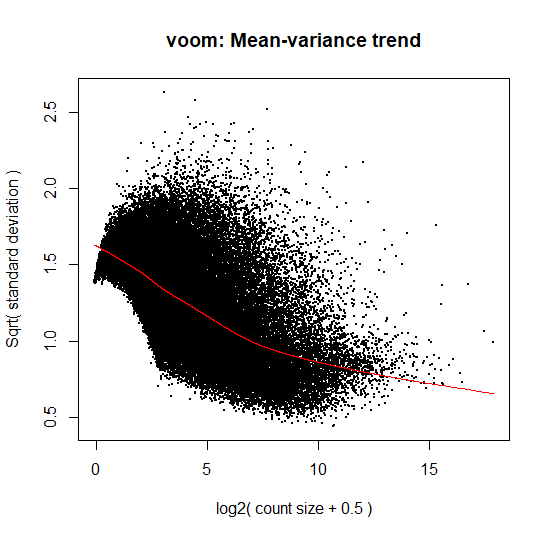Figure 5: Plot of Voom transformed data based on normalization factors

```    # Plotting the voom normalized counts of first twenty samples
boxplot(v\$E,xlab="",ylab="Voom normalized cpm",las=2)
abline(h=median(v\$E),col='red')
title("Boxplot of Voom normalised cpm")

```Figure 6: Box plot of Voom normalised cpm of first twenty samples

```    # Fitting the linear model and making contrasts
fit <- lmFit(v, design)
cont.matrix <- makeContrasts(infection=groupcovid-groupcontrol,levels=design)
fit.cont <- contrasts.fit(fit, cont.matrix)

# Calling the eBayes function to perform Bayes shrinkage on the variances
fit.cont <- eBayes(fit.cont)

# Summary of identified DEG’s
diff <- decideTests(fit.cont,adjust.method = 'BH',method = 'global',p.value = 0.01,lfc = 2)
summary(diff)

infection
Down         607
NotSig     62733
Up          1061

```

Note, we can observe the Number of Significant up and down-regulated genes in the table above.

```    # List of DEG’s whose p-value is < 0.01 and log fold change is > 2
sig <- topTable(fit.cont,adjust.method = "BH",p.value = 0.01,lfc = 2,number = 10000)
sig <- unique(sig)
sig <- setDT(sig,keep.rownames = 'ensembl_transcript_id')

```

After removing duplicates, the number of DEG’s reduced to 1613.

## Annotation of DEG’s

```mart <- useMart(biomart = "ensembl",dataset = "hsapiens_gene_ensembl")
results <- getBM(attributes = c("ensembl_gene_id","ensembl_transcript_id","hgnc_symbol",'description'),filters = "ensembl_transcript_id",values = sig\$ensembl_transcript_id,mart=mart)
final_annot <- merge(sig,results,all=TRUE,sort=FALSE)

```

Final annotation included 1613 DEG’s of 126 samples.

## Getting Up and Down-regulated DEG’s

```    up <- final_annot[final_annot\$logFC > 2,]
down <- final_annot[final_annot\$logFC < 2,]
up <- up[order(up\$logFC),]
print(up)

ensembl_transcript_id    logFC    AveExpr        t      P.Value    adj.P.Val           B ensembl_gene_id hgnc_symbol                                                                       description
1:       ENST00000354906 2.000701 -2.8160218 3.253365 1.460221e-03 9.951213e-03 -1.16577080 ENSG00000154305        MIA3             MIA SH3 domain ER export factor 3 [Source:HGNC Symbol;Acc:HGNC:24008]
2:       ENST00000518382 2.001017 -0.2068060 7.841295 1.550888e-12 2.171278e-09 17.15288614 ENSG00000104738        MCM4 minichromosome maintenance complex component 4 [Source:HGNC Symbol;Acc:HGNC:6947]
3:       ENST00000507960 2.002609 -0.8125808 3.537745 5.637281e-04 5.068281e-03 -0.34531675 ENSG00000002549        LAP3                      leucine aminopeptidase 3 [Source:HGNC Symbol;Acc:HGNC:18449]
4:       ENST00000517947 2.002836 -0.1276449 3.636493 3.998144e-04 3.929530e-03 -0.04873638 ENSG00000145907       G3BP1         G3BP stress granule assembly factor 1 [Source:HGNC Symbol;Acc:HGNC:30292]
5:       ENST00000485604 2.003443 -1.2675928 3.433180 8.052045e-04 6.541337e-03 -0.65184008 ENSG00000025293       PHF20                         PHD finger protein 20 [Source:HGNC Symbol;Acc:HGNC:16098]
---
1013:       ENST00000391614 5.216978  3.7936011 5.442061 2.599326e-07 1.364297e-05  6.60287022 ENSG00000132465      JCHAIN        joining chain of multimeric IgA and IgM [Source:HGNC Symbol;Acc:HGNC:5713]
1014:       ENST00000633299 5.367055  0.7144479 3.779857 2.399643e-04 2.687175e-03  0.36634975 ENSG00000282745     IGHV6-1              immunoglobulin heavy variable 6-1 [Source:HGNC Symbol;Acc:HGNC:5662]
1015:       ENST00000526285 5.980966  0.1975059 4.045652 8.986362e-05 1.278680e-03  1.17194772 ENSG00000173020        GRK2             G protein-coupled receptor kinase 2 [Source:HGNC Symbol;Acc:HGNC:289]
1016:       ENST00000612474 6.532706  0.7547895 3.975473 1.169861e-04 1.553727e-03  0.95335256 ENSG00000276491    IGHV4-34             immunoglobulin heavy variable 4-34 [Source:HGNC Symbol;Acc:HGNC:5650]
1017:       ENST00000510614 6.676199  1.8495323 4.201154 4.954081e-05 8.118264e-04  1.71871372 ENSG00000132465      JCHAIN        joining chain of multimeric IgA and IgM [Source:HGNC Symbol;Acc:HGNC:5713]

down <- down[order(down\$logFC),]
print(down)

ensembl_transcript_id     logFC    AveExpr         t      P.Value    adj.P.Val         B ensembl_gene_id hgnc_symbol                                                                                     description
1:       ENST00000587489 -5.700515  1.1134213 -8.823871 7.221547e-15 3.929708e-11 23.290907 ENSG00000184922       FMNL1                                                formin like 1 [Source:HGNC Symbol;Acc:HGNC:1212]
2:       ENST00000502702 -5.494837 -0.6258168 -7.880262 1.257789e-12 1.975680e-09 18.206728 ENSG00000071127        WDR1                                          WD repeat domain 1 [Source:HGNC Symbol;Acc:HGNC:12754]
3:       ENST00000511747 -5.445175 -0.8218833 -9.040463 2.166347e-15 1.550165e-11 24.201293 ENSG00000087266      SH3BP2                                SH3 domain binding protein 2 [Source:HGNC Symbol;Acc:HGNC:10825]
4:       ENST00000354161 -5.255502  1.2435909 -9.186280 9.600430e-16 8.832533e-12 25.241268 ENSG00000146828     SLC12A9                           solute carrier family 12 member 9 [Source:HGNC Symbol;Acc:HGNC:17435]
5:       ENST00000356909 -4.955808  2.1155965 -7.430572 1.381057e-11 1.046338e-08 16.009975 ENSG00000138964       PARVG                                                parvin gamma [Source:HGNC Symbol;Acc:HGNC:14654]
---
592:       ENST00000378543 -2.003592 -2.6714107 -4.394128 2.317643e-05 4.474176e-04  2.448801 ENSG00000162585      FAAP20                       FA core complex associated protein 20 [Source:HGNC Symbol;Acc:HGNC:26428]
593:       ENST00000533334 -2.003192  0.3647045 -5.782388 5.372443e-08 4.037231e-06  8.109110 ENSG00000105397        TYK2                                           tyrosine kinase 2 [Source:HGNC Symbol;Acc:HGNC:12440]
594:       ENST00000519276 -2.002662  2.7983683 -3.314567 1.195481e-03 8.632151e-03 -1.419526 ENSG00000164332      UBLCP1          ubiquitin like domain containing CTD phosphatase 1 [Source:HGNC Symbol;Acc:HGNC:28110]
595:       ENST00000533784 -2.000853 -0.9185426 -3.312018 1.205544e-03 8.674701e-03 -1.079109 ENSG00000158555       GDPD5 glycerophosphodiester phosphodiesterase domain containing 5 [Source:HGNC Symbol;Acc:HGNC:28804]
596:       ENST00000529867 -2.000117 -3.1892896 -4.244543 4.184459e-05 7.167110e-04  1.900040 ENSG00000167792      NDUFV1               NADH:ubiquinone oxidoreductase core subunit V1 [Source:HGNC Symbol;Acc:HGNC:7716]

```

Up-regulated DEG’s were 1017 and down-regulated were 596 in number.

## Functional analysis of DEG’s (Gene ontology)

Gene ontology analysis of the identified DEG’s was done using David functional analysis tools. Most of the DEG’s have been implicated in the host immunity and immune response pathways.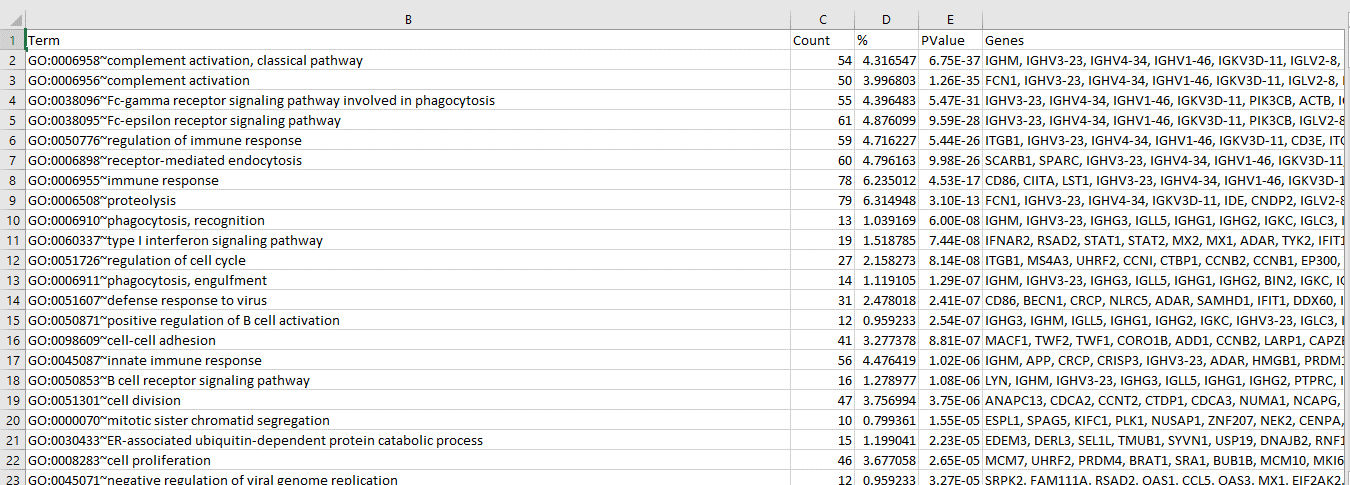Figure 7: Functional GO analysis of identified DEG’s

## Visualization of DEG’s

### Heat-map

```    diffgenes <- v\$E[diff[,1]!=0,]
mycol <- colorRampPalette(colors=c("green","white","blue"))(100)
hr <- hclust(as.dist(1-cor(t(diffgenes), method="pearson")), method="complete")
mycl <- cutree(hr, k=2)
mycolhc <- rainbow(length(unique(mycl)), start=0.1, end=0.9)
mycolhc <- mycolhc[as.vector(mycl)]
heatmap.2(diffgenes, Rowv=as.dendrogram(hr), Colv=NA,
col=mycol, scale="row", labRow=NA,
density.info="none", trace="none", RowSideColors=mycolhc,
cexRow=1, cexCol=1, margins=c(8,22))

```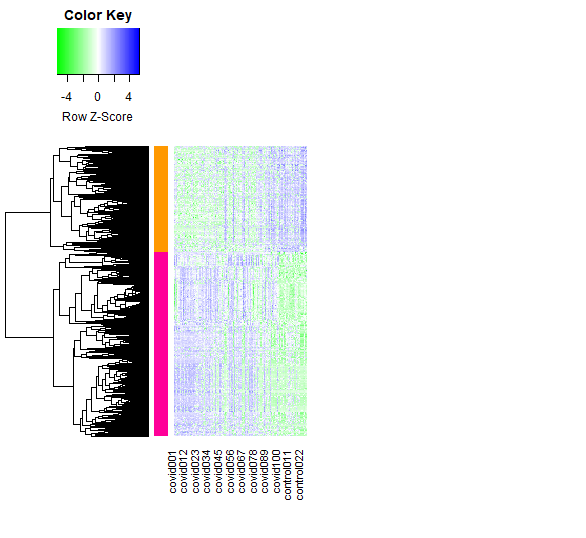Figure 8 : Heat-map of DEG’s between Covid and control samples

### Volcano plot and MDS plot

```    sample.cols <- c("limegreen", "purple")[group]
glXYPlot(x=fit.cont\$coef, y=fit.cont\$lod, xlab="logFC", ylab="logodds",status=diff,groups=group,sample.cols = sample.cols)
glMDPlot(fit.cont,status = diff[,"infection"], values=c(-1,1),cols = c("yellow","blue","magenta"))

```

Figure 9 : Volcano plot and MDS plot depicting DEG’s

```    # Getting counts of selected DEG's
colnames(counts) <- 'ensembl_transcript_id'
diff_counts2 <- merge(final_annot,counts,all=TRUE,sort=FALSE)
diff_counts2 <- slice(diff_counts2,(1:1613))
diff_counts2 <- diff_counts2 %>% select(-one_of(col))

# Transpose such that DEG's (hgnc_symbol) are columns and samples as rows
col_name <- diff_counts2\$hgnc_symbol
diff_counts_t <- as.data.frame(t(diff_counts2[,-1]))
colnames(diff_counts_t) <- col_name
table(duplicated(colnames(diff_counts_t)))
diff_counts_t <- diff_counts_t[,!duplicated(colnames(diff_counts_t))]
diff_counts_t\$Condition <- info\$Status
write.csv(diff_counts_t,'data.csv')

```

On removing duplicates, the final count of DEG’s was 1291.

## Feature selection of DEG’s

Now that the DEG’s were identified, feature selection was applied to reduce dimensionality.

1. Boruta algorithm was run on the above identified DEG’s (1291) with maximum runs of 500.

```    data <- read.csv("data.csv")
rownames(data) <- data\$X
data\$X <- NULL
bor <- Boruta(factor(Condition)~., data = data, maxRuns=500,doTrace=0)
boruta_df <- attStats(bor)
boruta_genes <- boruta_df[boruta_df\$decision=='Confirmed',]
par(mfrow=c(1,2))
plot(bor\$finalDecision, col=c('red','green','yellow'))
plotImpHistory(bor)

```

94 attributes were confirmed important by the Boruta algorithm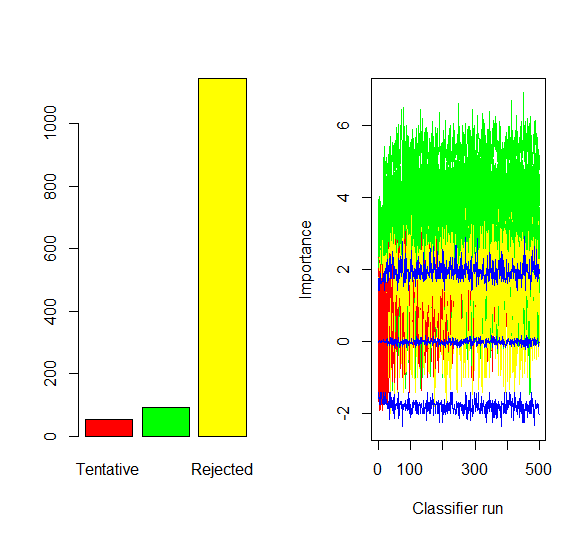Figure 10 : Plot of Final decision and ImpHistory of Boruta algorithm

2. Recursive feature elimination was run on the identified DEG’s using the function of random forest along with ten folds of repeated k-fold cross validation (using caret package).

```    x <- data[,1:1291]
y <- data[,1292]
y <- factor(y)
control <- rfeControl(functions = rfFuncs,method = 'repeatedcv',repeats = 10, number = 5)
res <- rfe(x,y,sizes=c(1:5,10,15,20),rfeControl=control)
rfe_genes <- data.frame(feature = row.names(varImp(res))[1:100],
importance = varImp(res)[1:100, 1])

# Plotting the importance of first ten DEG's
varimp_data2 <- data.frame(feature = row.names(varImp(res))[1:10],
importance = varImp(res)[1:10, 1])
ggplot(data = varimp_data2,
aes(x = reorder(feature, -importance), y = importance, fill = feature)) +
geom_bar(stat="identity") + labs(x = "Features", y = "Variable Importance") +
geom_text(aes(label = round(importance, 2)), vjust=1.6, color="white", size=4) +
theme_bw() + theme(legend.position = "none")

```

Top 100 attributes confirmed important by the RFE algorithm were chosen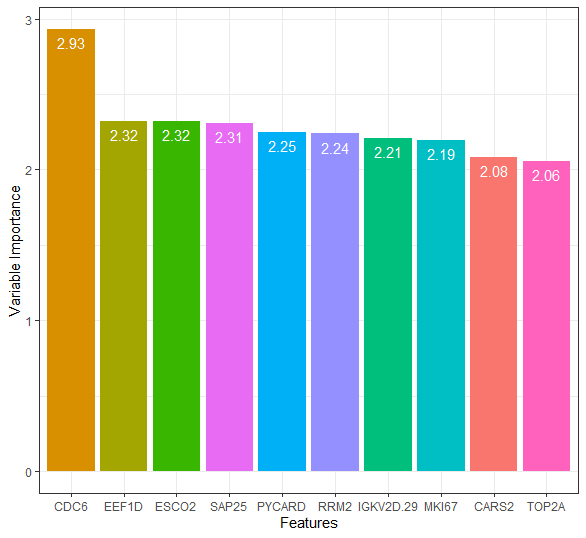Figure 10 : Plot of Final decision and ImpHistory of Boruta algorithm

## DEG’s commonly selected by Boruta and RFE

To find out the number of DEG’s that are common to both algorithms, Venn analysis was done.

```    boruta_genes\$genes <- rownames(boruta_genes)
ven <- venndetail(list(BORUTA=boruta_genes\$genes,RFE=rfe_genes\$feature))
plot(ven)

```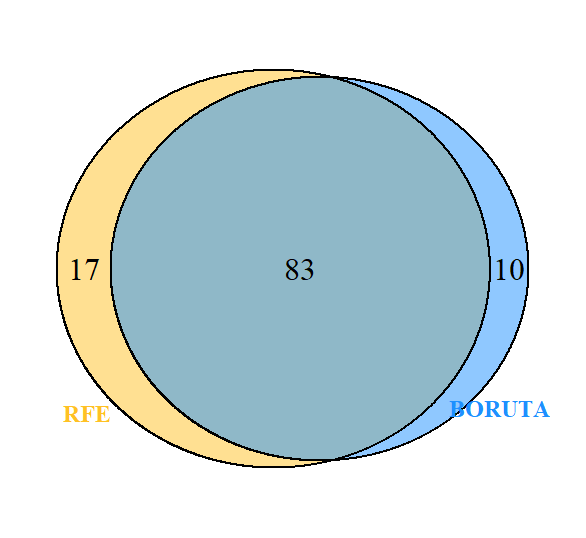Figure 11 : Venn diagram of genes common to Boruta and RFE

78 genes were commonly selected by both the algorithms. Next, we would build a random forest model with the selected features/genes for the classification of Covid-19.

## Random forest model with ten-fold cross validation

A random forest model with ten-fold cross-validation was built using the caret package. Four models were built so as to assess the importance of feature selection.

a. Model 1 with all identified DEG’s (no feature selection was applied)

```    # Data includes 1291 DEG's of 126 samples
data\$Condition <- factor(data\$Condition)
trControl <- trainControl(method = "cv",number = 10,search = "grid")
rf_crossval <- train(Condition~.,data = data,method = "rf",metric = "Accuracy",trControl = trControl)

```

b. Model 2 with genes selected by Boruta algorithm

```    # 94 DEG's selected by Boruta algorithm
# Sub setting Boruta selected genes from data
bor <- copy(rownames(boruta_genes))
bor_rf <- data[,c(bor)]
bor_rf\$Condition <- factor(info\$Status)
trControl <- trainControl(method = "cv",number = 10,search = "grid")
rf_crossval_boruta <- train(Condition~.,data = bor_rf,method = "rf",metric = "Accuracy",trControl = trControl)

```

c. Model 3 with genes selected by RFE algorithm

```    # 100 DEG's selected by RFE algorithm
# Sub setting RFE selected genes from data
rfe <- copy(rfe_genes\$feature)
rfe_rf <- data[,c(rfe)]
rfe_rf\$Condition <- factor(info\$Status)
trControl <- trainControl(method = "cv",number = 10,search = "grid")
rf_crossval_rfe <- train(Condition~.,data = rfe_rf,method = "rf",metric = "Accuracy",trControl = trControl)

```

d. Model 4 with genes common to both algorithms

```    # 78 DEG's selected by both algorithms
# Sub setting the shared genes from data
both <- intersect(boruta_genes\$genes,rfe_genes\$feature)
both <- copy(both)
shared <- data[,c(both)]
shared\$Condition <- factor(info\$Status)
trControl <- trainControl(method = "cv",number = 10,search = "grid")
rf_crossval_bor_rfe <- train(Condition~.,data = shared,method = "rf",metric = "Accuracy",trControl = trControl)

```

Sensitivity, Specificity and AUC score were obtained individually for each of the model by including class Probabilities and two class Summary in train Control and by changing the metric to “ROC”.

## Evaluation of Models

Performance of the models in classifying Covid-19 was assessed using the metrics: Accuracy, Sensitivity, Specificity and Area under ROC curve (AUC).

```    rf_crossval\$results

mtry  Accuracy     Kappa AccuracySD   KappaSD
1    2 0.9288462 0.7453651 0.04414863 0.1684855
2   50 0.9288462 0.7378999 0.05713165 0.2150076
3 1291 0.9057692 0.6510883 0.07028908 0.2894309

rf_crossval_boruta\$results

mtry  Accuracy     Kappa AccuracySD   KappaSD
1    2 0.9685897 0.8865753 0.05443059 0.2025097
2   47 0.9294872 0.7565949 0.06757004 0.2351235
3   93 0.9141026 0.7018150 0.07639897 0.2613480

rf_crossval_rfe\$results

mtry  Accuracy     Kappa AccuracySD   KappaSD
1    2 0.9756410 0.9129717 0.03926046 0.1443566
2   51 0.9365385 0.7723934 0.06313009 0.2315501
3  100 0.9442308 0.8043868 0.05506440 0.1996204

rf_crossval_bor_rfe\$results

mtry  Accuracy     Kappa AccuracySD   KappaSD
1    2 0.9615385 0.8830818 0.05439283 0.1604058
2   42 0.9294872 0.7566853 0.05701566 0.1984533
3   83 0.9294872 0.7566853 0.05701566 0.1984533

```Figure 12 : Performance metrics of the four models

As evident from figure 12, Models 2 & 3 with roughly 90-100 predictors/DEG’s performed better than Model 1 with 1291 predictors.  Model 4, with just 78 predictors achieved an outstanding AUC score of 1.00. Thus, feature selection efficiently reduced the dimensionality without compromising the variance in the data and further strengthened the overall performance of the machine learning model.

The relative feature importance of the top 20 DEG’s commonly selected by both algorithms is depicted below.

```    plot(varImp(rf_crossval_bor_rfe),col=c('blue','red','green','yellow'),top=20)

```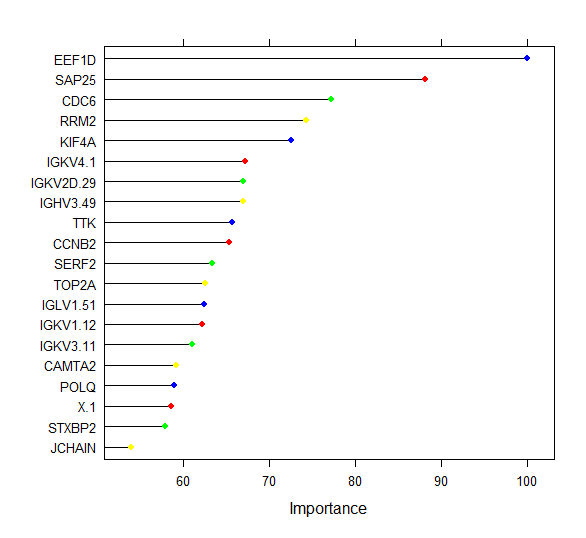Figure 13 : Ranking of the Top 20 DEG’s

## Conclusion

In conclusion, it could be highlighted that machine learning based feature selection plays a vital role in identifying key DEG’s from the high dimensional RNA-Seq data which further helps in better understanding of the pathologic condition and to differentiate the diseased from the healthy. It is also noteworthy to mention that the selected DEG’s can serve as potential biomarkers for diagnostic and therapeutic purposes.